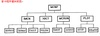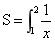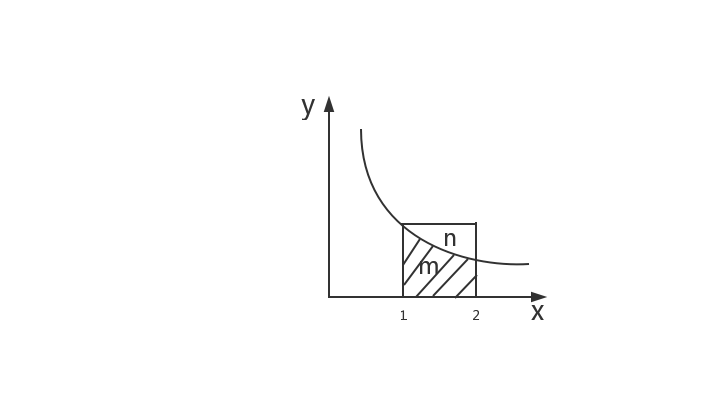Monte Carlo method

• 蒙特卡洛算法详解，举例为用蒙特卡洛算法求解pi，及其相关知识
• 利用MATLAB对蒙特卡洛算法进行仿真实验 实时绘出粒子群中心与实际位置轨迹 并能实时绘制误差信息等
• 蒙特卡洛算法，带有案例。MATLAB语言编写。VHS couette DSMC for couette flow
• python随机数、MCMC蒙特卡洛算法
MCMC算法
蒙特卡罗方法又称统计模拟法、随机抽样技术，是一种随机模拟方法，以概率和统计理论方法为基础的一种计算方法，是使用随机数（或更常见的伪随机数）来解决很多计算问题的方法。 当所求解问题是某种随机事件出现的概率，或者是某个随机变量的期望值时，通过某种“实验”的方法，以这种事件出现的频率估计这一随机事件的概率，或者得到这个随机变量的某些数字特征，并将其作为问题的解。 个人浅见：随机数模拟+概率论=MCMC
示例：蒙特卡洛算法求积分
首先考虑如下积分求如上积分就是求下图中阴影面积。利用蒙特卡洛算法，随机模拟正方形中的点（Xi,Yi），其总数为n,若1/Xi大于等于Yi，则表示点（Xi，Yi）位于阴影面积内，满足这一条件的点个数为m。由概率论可知， S=m/n*(2-1)*(1-0)
import random
max=10000000
def getPoint():
x=random.uniform(1.0,2.0)
y=random.uniform(0.0,1.0)
p=(x,y)
return p
def getResult():
m=0
n=max
for i in range(n):
p=getPoint()
if(1/p>=p):
m+=1
return m/n*(2-1)*(1-0)
print(getResult())


Python中随机数的生成：
import random
random.randint(1,10) #产生1-10的一个随机整数
random.random()  #产生[0,1)中的一个随机浮点数
random.uniform(1.1,3.2) #生成1.1到3.2间隔中的一个随机浮点数，区间可以不是整数
random.choice('beautiful') #从序列中随机选一个元素
random.randrange(1,100,2) #生成1到100间隔为2的一个元素
a=[1,2,3,4,5]
random.shuffle(a)  #将序列a的元素打乱


展开全文python随机数
• 蒙特卡洛算法Matlab程序代码，个人搜集，希望有所帮助...